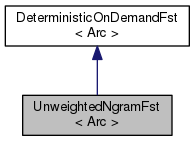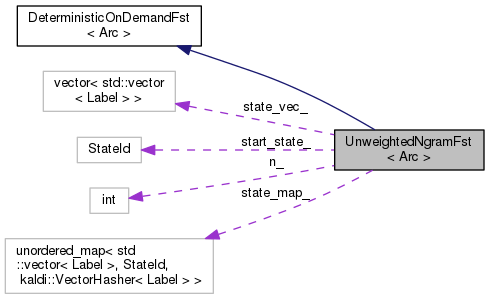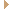UnweightedNgramFst< Arc > Class Template Reference

The class UnweightedNgramFst is a DeterministicOnDemandFst whose states encode an n-gram history. More...

`#include <deterministic-fst.h>`

Inheritance diagram for UnweightedNgramFst< Arc >:[legend]
Collaboration diagram for UnweightedNgramFst< Arc >:[legend]

## Public Types

typedef Arc::Weight Weight

typedef Arc::StateId StateId

typedef Arc::Label LabelPublic Types inherited from DeterministicOnDemandFst< Arc >
typedef Arc::StateId StateId

typedef Arc::Weight Weight

typedef Arc::Label Label

## Public Member Functions

UnweightedNgramFst (int n)

StateId Start ()

Weight Final (StateId s)

bool GetArc (StateId s, Label ilabel, Arc *oarc)
Note: ilabel must not be epsilon. More...Public Member Functions inherited from DeterministicOnDemandFst< Arc >
virtual ~DeterministicOnDemandFst ()

## Private Types

typedef unordered_map< std::vector< Label >, StateId, kaldi::VectorHasher< Label > > MapType

## Private Attributes

int n_

MapType state_map_

StateId start_state_

std::vector< std::vector< Label > > state_vec_

## Detailed Description

### template<class Arc> class fst::UnweightedNgramFst< Arc >

The class UnweightedNgramFst is a DeterministicOnDemandFst whose states encode an n-gram history.

Conceptually, for n-gram order n and k labels, the FST is an unweighted acceptor with about k^(n-1) states (ignoring end effects). However, the FST is created on demand and doesn't need the label vocabulary; GetArc matches on any input label. This class is primarily used together with ComposeDeterministicOnDemandFst to expand the n-gram history of lattices, ensuring that each arc has a sufficiently long unique word history.

Definition at line 173 of file deterministic-fst.h.

## ◆ Label

 typedef Arc::Label Label

Definition at line 177 of file deterministic-fst.h.

## ◆ MapType

 typedef unordered_map, StateId, kaldi::VectorHasher
private

Definition at line 189 of file deterministic-fst.h.

## ◆ StateId

 typedef Arc::StateId StateId

Definition at line 176 of file deterministic-fst.h.

## ◆ Weight

 typedef Arc::Weight Weight

Definition at line 175 of file deterministic-fst.h.

## ◆ UnweightedNgramFst()

 UnweightedNgramFst ( int n )

Definition at line 89 of file deterministic-fst-inl.h.

89  : n_(n) {
90  // Starting state is an empty vector
91  std::vector<Label> start_state;
92  state_vec_.push_back(start_state);
93  start_state_ = 0;
94  state_map_[start_state] = 0;
95 }
std::vector< std::vector< Label > > state_vec_
struct rnnlm::@11::@12 n

## ◆ Final()

 Arc::Weight Final ( StateId s )
virtual

Implements DeterministicOnDemandFst< Arc >.

Definition at line 130 of file deterministic-fst-inl.h.

References KALDI_ASSERT, and UnweightedNgramFst< Arc >::state_vec_.

130  {
131  KALDI_ASSERT(state < static_cast<StateId>(state_vec_.size()));
132  return Weight::One();
133 }
std::vector< std::vector< Label > > state_vec_
#define KALDI_ASSERT(cond)
Definition: kaldi-error.h:185

## ◆ GetArc()

 bool GetArc ( StateId s, Label ilabel, Arc * oarc )
virtual

Note: ilabel must not be epsilon.

Implements DeterministicOnDemandFst< Arc >.

Definition at line 98 of file deterministic-fst-inl.h.

99  {
100
101  // The state ids increment with each state we encounter.
102  // if the assert fails, then we are trying to access
103  // unseen states that are not immediately traversable.
104  KALDI_ASSERT(static_cast<size_t>(s) < state_vec_.size());
105  std::vector<Label> seq = state_vec_[s];
106  // Update state info.
107  seq.push_back(ilabel);
108  if (seq.size() > n_-1) {
109  // Remove oldest word in the history.
110  seq.erase(seq.begin());
111  }
112  std::pair<const std::vector<Label>, StateId> new_state(
113  seq,
114  static_cast<Label>(state_vec_.size()));
115  // Now get state id for destination state.
116  typedef typename MapType::iterator IterType;
117  std::pair<IterType, bool> result = state_map_.insert(new_state);
118  if (result.second == true) {
119  state_vec_.push_back(seq);
120  }
121  oarc->weight = Weight::One(); // Because the FST is unweightd.
122  oarc->ilabel = ilabel;
123  oarc->olabel = ilabel;
124  oarc->nextstate = result.first->second; // The next state id.
125  // All arcs can be matched.
126  return true;
127 }
std::vector< std::vector< Label > > state_vec_
#define KALDI_ASSERT(cond)
Definition: kaldi-error.h:185

## ◆ Start()

 StateId Start ( )
inlinevirtual

Implements DeterministicOnDemandFst< Arc >.

Definition at line 181 of file deterministic-fst.h.

181 { return start_state_; };

## ◆ n_

 int n_
private

Definition at line 191 of file deterministic-fst.h.

Referenced by UnweightedNgramFst< Arc >::GetArc().

## ◆ start_state_

 StateId start_state_
private

Definition at line 193 of file deterministic-fst.h.

Referenced by UnweightedNgramFst< Arc >::UnweightedNgramFst().

## ◆ state_map_

 MapType state_map_
private

Definition at line 192 of file deterministic-fst.h.

## ◆ state_vec_

 std::vector > state_vec_
private

Definition at line 195 of file deterministic-fst.h.

The documentation for this class was generated from the following files: